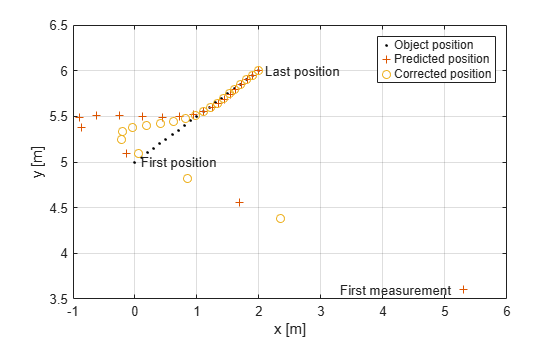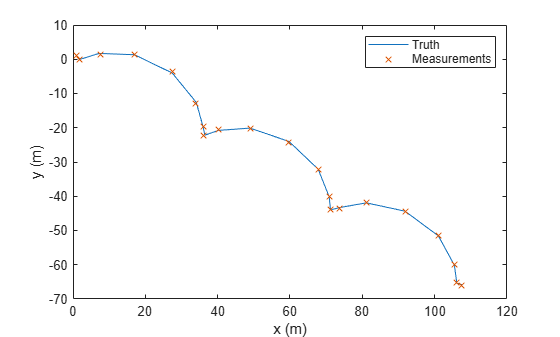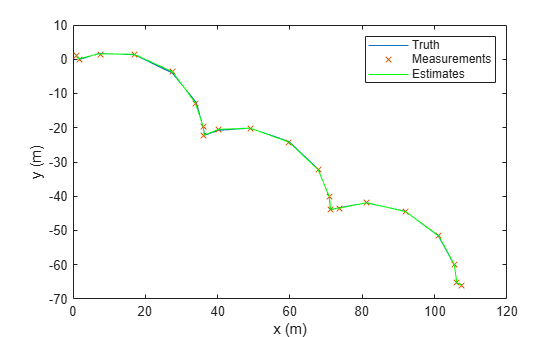# predict

Predict state and state estimation error covariance of linear Kalman filter

Since R2021a

## Syntax

``[xpred,Ppred] = predict(filter)``
``[xpred,Ppred] = predict(filter,dt)``
``[xpred,Ppred] = predict(filter)``
``[xpred,Ppred] = predict(filter,A)``
``[xpred,Ppred] = predict(filter,A,Q)``
``[xpred,Ppred] = predict(filter,u)``
``[xpred,Ppred] = predict(filter,u,A,B)``
``[xpred,Ppred] = predict(filter,u,A,B,Q)``

## Description

### Syntaxes for Predefined Motion Model

Use these syntaxes if you specify a predefined motion model in the `MotionModel` property of the `trackingKF` object.

````[xpred,Ppred] = predict(filter)` returns the predicted state `xpred` and the predicted state estimation error covariance `Ppred` after one second using the motion model specified in the `filter`. The predicted values overwrite the internal state and state estimation error covariance of the `filter`.```

example

````[xpred,Ppred] = predict(filter,dt)` predicts the state and state estimation error covariance at the specified time step `dt`.```

### Syntaxes for Custom Motion Model Without Control Input

Use these syntaxes if you specify the `MotionModel` property as `"Custom"` and do not use control inputs.

````[xpred,Ppred] = predict(filter)` returns the predicted state `xpred` and the predicted state estimation error covariance `Ppred` using the state transition matrix specified in the `StateTransitionModel` property of the `filter`. The predicted values overwrite the internal state and state estimation error covariance of the `filter`.```
````[xpred,Ppred] = predict(filter,A)` specifies the state transition model `A`. Use this syntax when the state transition model is time-varying.```
````[xpred,Ppred] = predict(filter,A,Q)` specifies the state transition model `A` and the process noise covariance `Q`. Use this syntax when the state transition model and the process noise are time-varying.```

### Syntaxes for Custom Motion Model with Control Input

Use these syntaxes if you specify the `MotionModel` property as `"Custom"` and use control inputs.

example

````[xpred,Ppred] = predict(filter,u)` returns the predicted state `xpred` and the predicted state estimation error covariance `Ppred` using the state transition model specified in the `StateTransitionModel` property of the `filter` and a control input `u`.```
````[xpred,Ppred] = predict(filter,u,A,B)` specifies the force or control input `u`, the state transition model `A`, and the control model `B`. Use this syntax when the state transition model and control model are time-varying.```
````[xpred,Ppred] = predict(filter,u,A,B,Q)` specifies the force or control input `u`, the state transition model `A`, the control model `B`, and the process noise covariance `Q`. Use this syntax when the state transition model, control model, and process noise are time-varying.```

## Examples

collapse all

Create a linear Kalman filter that uses a 2D constant velocity motion model. Assume that the measurement consists of the xy-location of the object.

Specify the initial state estimate to have zero velocity.

```x = 5.3; y = 3.6; initialState = [x;0;y;0]; KF = trackingKF('MotionModel','2D Constant Velocity','State',initialState);```

Create measured positions for the object on a constant-velocity trajectory.

```vx = 0.2; vy = 0.1; T = 0.5; pos = [0:vx*T:2; 5:vy*T:6]';```

Predict and correct the state of the object.

```for k = 1:size(pos,1) pstates(k,:) = predict(KF,T); cstates(k,:) = correct(KF,pos(k,:)); end```

Plot the tracks.

```plot(pos(:,1),pos(:,2),"k.",pstates(:,1),pstates(:,3),"+", ... cstates(:,1),cstates(:,3),"o") xlabel("x [m]") ylabel("y [m]") grid xt = [x-2, pos(1,1)+0.1, pos(end,1)+0.1]; yt = [y, pos(1,2), pos(end,2)]; text(xt,yt,["First measurement","First position","Last position"]) legend("Object position","Predicted position","Corrected position")```Specify a simulation time of 10 seconds with a time step of 1 second.

```rng(2021) % For repeatable results simulationTime = 20; dt = 1; tspan = 0:dt:simulationTime; steps = length(tspan);```

Specify the motion model as a 2-D constant velocity model with a state of [`x`; `vx`; `y`; `vy`]. The measurement is [`x`; `y`].

```A1D = [1 dt; 0 1]; A = kron(eye(2),A1D) % State transiton model```
```A = 4×4 1 1 0 0 0 1 0 0 0 0 1 1 0 0 0 1 ```
```H1D = [1 0]; H = kron(eye(2),H1D) % Measurement model ```
```H = 2×4 1 0 0 0 0 0 1 0 ```
```sigma = 0.2; R = sigma^2*eye(2); % Measurement noise covariance```

Specify a control model matrix.

```B1D = [0; 1]; B = kron(eye(2),B1D) % Control model matrix```
```B = 4×2 0 0 1 0 0 0 0 1 ```

Assume the control inputs are sinusoidal on the velocity components, `vx` and `vy`.

```gain = 5; Ux = gain*sin(tspan(2:end)); Uy = gain*cos(tspan(2:end)); U =[Ux; Uy]; % Control inputs```

Assuming the true initial state is [`1 1 1 -1`], simulate the system to obtain true states and measurements.

```initialState = [1 1 1 -1]'; % [m m/s m m/s] trueStates = NaN(4,steps); trueStates(:,1) = initialState; for i=2:steps trueStates(:,i) = A*trueStates(:,i-1) + B*U(:,i-1); end measurements = H*trueStates + chol(R)*randn(2,steps);```

Visualize the true trajectory and the measurements.

```figure plot(trueStates(1,:),trueStates(3,:),"DisplayName","Truth") hold on plot(measurements(1,:),measurements(2,:),"x","DisplayName","Measurements") xlabel("x (m)") ylabel("y (m)") legend```Create a `trackingKF` filter with a custom motion model. Enable the control input by specifying the control model. Specify the initial state in the filter based on the first measurement.

```initialFilterState = [measurements(1,1); 0; measurements(2,1); 0]; filter = trackingKF("MotionModel","Custom", ... "StateTransitionModel",A, ... "MeasurementModel",H, ... "ControlModel",B, ... "State",initialFilterState);```

Estimate states by using the `predict` and `correct` object functions.

```estimateStates = NaN(4,steps); estimateStates(:,1) = initialFilterState; for i = 2:steps predict(filter,U(:,i-1)); estimateStates(:,i) = correct(filter,measurements(:,i)); end```

Visualize the state estimates.

`plot(estimateStates(1,:),estimateStates(3,:),"g","DisplayName","Estimates");`## Input Arguments

collapse all

Linear Kalman filter for object tracking, specified as a `trackingKF` object.

Time step, specified as a positive scalar. Units are in seconds.

State transition model, specified as a real-valued M-by-M matrix, where M is the size of the state vector.

Covariance of process noise, specified as a nonnegative scalar, a positive-semidefinite D-by-D matrix, or a positive-semidefinite M-by-M matrix.

• When the `MotionModel` property of the `filter` is specified as one of the predefined motion models, specify `Q` as a positive-semidefinite D-by-D matrix, where D is the number of dimensions of the target motion. For example, D = 2 for the `"2D Constant Velocity"` or the `"2D Constant Acceleration"` motion model.

In this case, if you specify `Q` as a nonnegative scalar, then the scalar extends to the diagonal elements of a diagonal covariance matrix, whose size is D-by-D .

• When the `MotionModel` property of the `filter` is specified as `"Custom"`, specify `Q` as a positive-semidefinite M-by-M matrix, where M is the size of the filter state. For example, M = 6 if you customize a 3-D motion model in which the state is (x, vx, y, vy, z, vz).

In this case, if you specify `Q` as a nonnegative scalar, then the scalar extends to the diagonal elements of a diagonal covariance matrix, whose size is M-by-M.

Control vector, specified as a real-valued L-element vector.

Control model, specified as a real-valued M-by-L matrix. M is the size of the state vector. L is the number of independent controls.

## Output Arguments

collapse all

Predicted state, returned as a real-valued M-element vector. The predicted state represents the deducible estimate of the state vector, propagated from the previous state using the state transition and control models.

Predicted state covariance matrix, returned as a real-valued M-by-M matrix. M is the size of the state vector. The predicted state covariance matrix represents the deducible estimate of the covariance matrix vector. The filter propagates the covariance matrix from the previous estimate.

## Version History

Introduced in R2021a